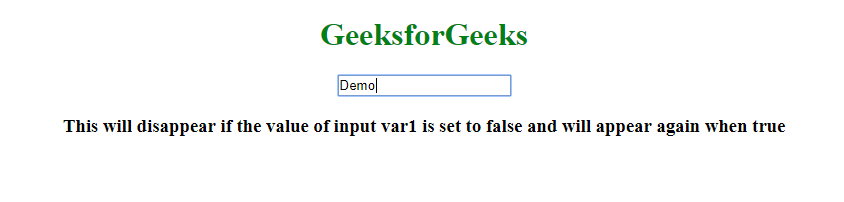# What is the difference between ng-if and data-ng-if directives ?

The ng-if is a directive in AngularJS which is used to remove the HTML element if the value of the expression or variable is false, unlike ng-hide which just hides the HTML element from the DOM.

Syntax:

`<element angular_directive=expression> Contents... </element>`

There are few other options which behave like ng-if. There is no difference among them in functionality wise.

• ng:if
• ng_if
• x-ng-if
• data-ng-if

Note: The best practice is to use ng-if only.

The reason behind why these options come into the picture is that in AngularJS we refer to the directive using camel case (example:ngIf) but when we use it in HTML since HTML is case insensitive we use a dash-delimited form (example:ng-if) or other delimiters as mentioned in the list above. So the AngularJS normalizes (It means it converts the delimiter form into camelcase.) the element’s tag and figures out to which directive does the element belong.

Example 1: This example uses “data-ng-if” directive.

 ` ` `<``html``> ` ` `  `<``head``> ` `    ``<``title``> ` `        ``What is the difference between ng-if ` `        ``and data-ng-if directives ? ` `    `` ` `     `  `    ``<``script` `src``= ` `"https://ajax.googleapis.com/ajax/libs/angularjs/1.6.9/angular.min.js"``> ` `    `` ` ` ` ` `  `<``body` `ng-app``=``""``> ` `    ``<``center``> ` `        ``<``h1` `style``=``"color:green"``> ` `            ``GeeksforGeeks ` `        `` ` ` `  `        ``<``input` `ng-model``=``"var1"``> ` ` `  `        ``<``div` `data-ng-if``=``"var1"``> ` `            ``<``h3``> ` `                ``This will disappear if the value of ` `                ``input var1 is set to false and will  ` `                ``appear again when true ` `            `` ` `        `` ` `    `` ` ` ` ` `  ` `

Output:Example 2: This example uses “ng-if” directive.

 ` ` `<``html``> ` ` `  `<``head``> ` `    ``<``title``> ` `        ``What is the difference between ng-if ` `        ``and data-ng-if directives ? ` `    `` ` `     `  `    ``<``script` `src``= ` `"https://ajax.googleapis.com/ajax/libs/angularjs/1.6.9/angular.min.js"``> ` `    `` ` ` ` ` `  `<``body` `ng-app``=``""``> ` `    ``<``center``> ` `        ``<``h1` `style``=``"color:green"``> ` `            ``GeeksforGeeks ` `        `` ` ` `  `        ``<``input` `ng-model``=``"var1"``> ` ` `  `        ``<``div` `ng-if``=``"var1"``> ` `            ``<``h3``> ` `                ``This will disappear if the value of ` `                ``input var1 is set to false and will  ` `                ``appear again when true ` `            `` ` `        `` ` `    `` ` ` ` ` `  ` `

Output:My Personal Notes arrow_drop_upCheck out this Author's contributed articles.

If you like GeeksforGeeks and would like to contribute, you can also write an article using contribute.geeksforgeeks.org or mail your article to contribute@geeksforgeeks.org. See your article appearing on the GeeksforGeeks main page and help other Geeks.

Please Improve this article if you find anything incorrect by clicking on the "Improve Article" button below.

Article Tags :

Be the First to upvote.

Please write to us at contribute@geeksforgeeks.org to report any issue with the above content.